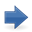### A Model of the Relationship between the Interest Rate and the Profit Rate

CSWP 55 (June 2022)

Author Riccardo Zolea

Keywords bank profitability; inetrest rate; price equation; Marx; finance

JEL E11; E43; G21

In this paper we investigate the relationship between interest rate and profit rate. Considering that the bank must obtain on the invested capital a profit rate at least equal to the normal one, the bank interest rate can be calculated as the price of the bank output, i.e. the loan. The profit rate thus determines the interest rate, through the instrument of the price equation. Central to this study is the analysis of the best way to imagine such a price equation, considering the role of the central bank.
We then move on to study the structure of interest rates and their relationship to the profit rate. Finally, by introducing the hypothesis of a bank profit rate permanently higher than the normal one, we confirm and better explain from an analytical point of view some insights expressed by Marx in Book III of Capital.Download the paper